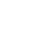# Henry's Law Constants

### Rolf Sander

Atmospheric Chemistry Division

Max-Planck Institute for Chemistry
Mainz, Germany

## Errata

#### Contact, Impressum, Acknowledgements

When referring to the compilation of Henry's Law Constants, please cite this publication:

R. Sander: Compilation of Henry's law constants (version 4.0) for water as solvent, Atmos. Chem. Phys., 15, 4399-4981 (2015), doi:10.5194/acp-15-4399-2015

# Converting Henry's Law Constants

## Your input: $Hscp$ = 1 $mol/(m3⋅Pa)$ (aq-concentration / partial pressure)

### Conversion to Henry's law solubility constants $Hs$ yields:

 $Hscp$ = 1 $mol/(m3⋅Pa)$ (aq-concentration / partial pressure) $Hscp$ = 101.325 $M/atm$ (aq-concentration / partial pressure) $Hscc$ = 2478.96  (aq-concentration / gas-concentration) $Hsbp$ = 0.00100301 $mol/(kg⋅Pa)$ (molality / partial pressure) $Hsbp$ = 101.63 $mol/(kg⋅atm)$ (molality / partial pressure) $Hsxp$ = 1.83089 $atm-1$ (molar fraction / partial pressure) $α$ = 2271.1  (Bunsen coefficient)

### Conversion to Henry's law volatility constants $Hv$ yields:

 $Hvpx$ = 0.546182 $atm$ (partial pressure / molar fraction) $Hvpc$ = 1 $m3⋅Pa/mol$ (partial pressure / aq-concentration) $Hvpc$ = 9.86923e-06 $m3⋅atm/mol$ (partial pressure / aq-concentration) $Hvcc$ = 0.000403395  (gas-concentration / aq-concentration)

All conversions are calculated at a temperature of T = 298.15 K and a solution density of ϱ = 997 kg/m3, using the conversion factors from Sander (2015) as shown in the following tables.

Table 1: Conversion factors between several Henry's law solubility constants $Hs$.

 $Hscp$ = ... $molm3⋅Pa$ $Hscp$ = ... $Matm$ $Hscc$ = ... $Hsbp$ = ... $molkg⋅Pa$ $Hsbp$ = ... $molkg⋅atm$ $Hsxp$ = ... $1atm$ α = ... $Hscp$ = 1 $molm3⋅Pa$ 1.00000 101.325 2478.96 1.00301E-3 101.630 1.83089 2271.10 $Hscp$ = 1 $Matm$ 9.86923E-3 1.00000 24.4654 9.89893E-6 1.00301 0.0180695 22.4140 $Hscc$ = 1 4.03395E-4 0.0408740 1.00000 4.04609E-7 0.0409970 7.38573E-4 0.916150 $Hsbp$ = 1 $molkg⋅Pa$ 997.000 1.01021E5 2.47152E6 1.00000 1.01325E5 1825.40 2.26428E6 $Hsbp$ = 1 $molkg⋅atm$ 9.83962E-3 0.997000 24.3920 9.86923E-6 1.00000 0.0180153 22.3467 $Hsxp$ = 1 $1atm$ 0.546182 55.3419 1353.96 5.47826E-4 55.5084 1.00000 1240.43 α = 1 4.40316E-4 0.0446150 1.09152 4.41641E-7 0.0447493 8.06171E-4 1.00000

Table 2: Conversion factors between several Henry's law volatility constants $Hv$.

 $Hvpx$ = ... $atm$ $Hvpc$ = ... $m3⋅Pamol$ $Hvpc$ = ... $m3⋅atmmol$ $Hvcc$ = ... $Hvpx$ = 1 $atm$ 1.00000 1.83089 1.80695E-5 7.38573E-4 $Hvpc$ = 1 $m3⋅Pamol$ 0.546182 1.00000 9.86923E-6 4.03395E-4 $Hvpc$ = 1 $m3⋅atmmol$ 55341.9 1.01325E5 1.00000 40.8740 $Hvcc$ = 1 1353.96 2478.96 0.0244654 1.00000

Table 3: Products of Henry's law solubility constants $Hs$ and Henry's law volatility constants $Hv$. For example, if $Hvpx$ = 11.1 atm, then $Hsbp$ 5 mol/(kg atm) because 11.1 * 5 55.5084.

 $Hscpmol/(m3⋅Pa)$ $HscpM/atm$ $Hscc1$ $Hsbpmol/(kg⋅Pa)$ $Hsbpmol/(kg⋅atm)$ $Hsxp1/atm$ $α1$ $Hvpxatm$ 0.546182 55.3419 1353.96 5.47826E-4 55.5084 1.00000 1240.43 $Hvpcm3⋅Pa/mol$ 1.00000 101.325 2478.96 1.00301E-3 101.630 1.83089 2271.10 $Hvpcm3⋅atm/mol$ 9.86923E-6 1.00000E-3 0.0244654 9.89893E-9 1.00301E-3 1.80695E-5 0.0224140 $Hvcc1$ 4.03395E-4 0.0408740 1.00000 4.04609E-7 0.0409970 7.38573E-4 0.916150

# * * *

## Convert Henry's Law Constants

Convert: mol/(m3*Pa) (Hscp) M/atm (Hscp) 1/atm (Hsxp) mol/(kg*Pa) (Hsbp) mol/(kg*atm) (Hsbp) (dimensionless aq/g) (Hscc) m3*Pa/mol (Hvpc) m3*atm/mol (Hvpc) atm (Hvpx) (dimensionless g/aq) (Hvcc) (dimensionless aq/g) (alpha)